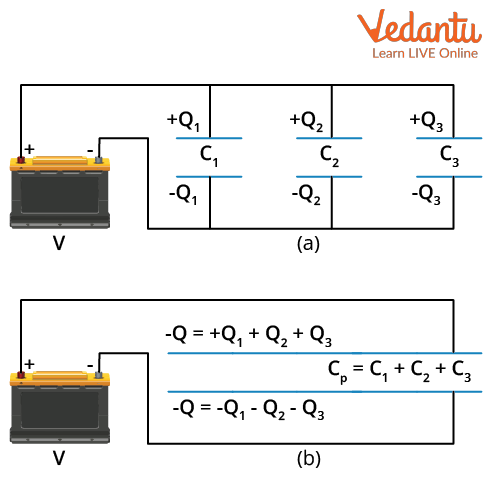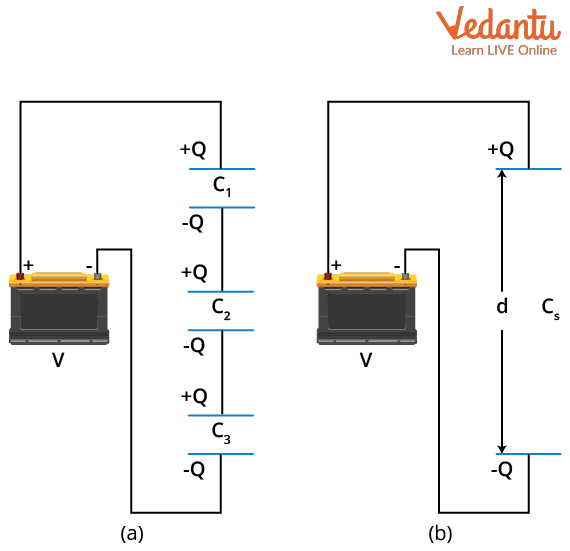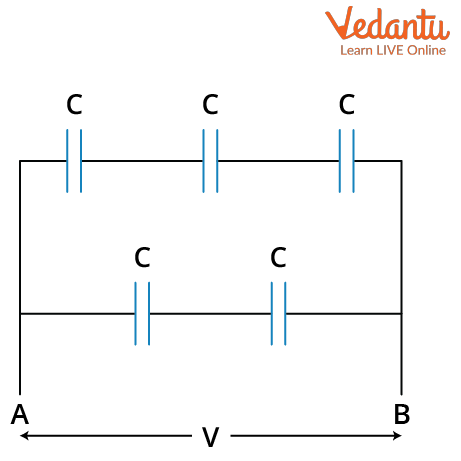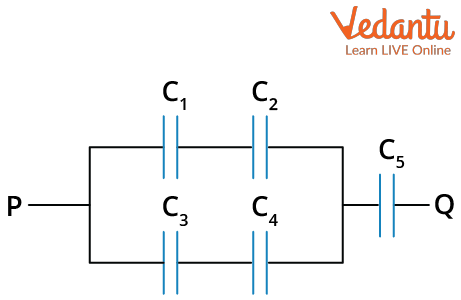Courses
Courses for Kids
Free study material
Offline Centres
More

# Combination of Capacitors - In Parallel and Series for JEELast updated date: 27th Nov 2023
Total views: 21k
Views today: 0.21k## Why is a Combination of Capacitors Needed?

One could wonder why combinations of capacitors are needed? The combination of capacitors is fairly similar to that of the combination of cells that are used in everyday life. Suppose one needs an 8V DC supply and has four 2V electric cells, then we can quickly connect these cells to get the required supply. The cells would be connected in series and then the desired 8V power supply can be obtained. Similarly, we also use a combination of capacitors to get the required capacities for a variety of uses.

Capacitors are interesting devices to study. By producing an electric field between two conductors, they allow us to store electrical energy inside a circuit. They are found in practically every electronic gadget and are essential to the operation of many of them. Capacitors are designed to get a fixed capacity and each capacitor has its unique capacity. So to get an effective capacitance for a particular purpose, a combination of capacitors can be used.

When we are required to increase the effective capacitance in the circuit we are working on, we can always add a capacitor in parallel to the already existing capacitor. Similarly, when the effective capacitance is to be decreased, one can add a capacitor in series with the existing capacitance.

## How are Capacitors Connected?

The capacitance changes brought upon in the circuit by adding another capacitor depend on the way the capacitors are connected. Capacitor combinations can be made in a variety of ways depending on the person who is making the circuit. The combinations are then connected in a circuit to a battery with a potential difference V. Due to this potential difference, a charge Q is introduced between the plates of the capacitor. The equivalent capacitance between the two plates of a capacitor is given as,

$C=\dfrac{Q}{V}$

The variety of ways that combinations of capacitors can be made is divided into two methods. They are:

• Parallel Combination

• Series Combination

These methods have different equivalent capacitance and we will be looking at them in detail.

## Parallel Combination of Capacitors

When the two terminals of one capacitor are connected to each terminal of another capacitor, the capacitors are said to be in a parallel combination. The voltage is the same across all the capacitors that are linked in parallel. This is the key to finding out the effective capacitance of the combination.

Consider the case of three capacitors connected in a parallel combination. If each capacitor has a capacitance of C1, C2, and C3, the parallel equivalent capacitance is CP.

Figure (a) shows a parallel configuration of three capacitors, with one plate of each capacitor connected to one side of the circuit and the other plates to the other side of the circuit.Image: Parallel combination of capacitors

Since they are connected in parallel, they have the same voltage across their plates. But each capacitor can store different charges between their plates depending on their capacity. To find the equivalent capacitance of the combination, we first define the total charge Q stored by the combination. This is given as,

$Q=Q_{1}+Q_{2}+Q_{3}$

Now, since we know that the voltage across all of them is the same, using the formula for capacitance, Q=CV we can write the formula for the charge of each capacitor as,

\begin{align} &Q_{1}=C_{1} V \\ &Q_{2}=C_{2} V \\ &Q_{3}=C_{3} V \end{align}

The total charge can be written as,

$C_{P} V=C_{1} V+C_{2} V+C_{3} V$

Simplifying this gives,

$C_{P}=C_{1}+C_{2}+C_{3}$

This equation can be generalized for n capacitors. The equivalent capacitance will be,

$C_{P}=C_{1}+C_{2}+C_{3}+\ldots+C_{n}$

This is the parallel capacitor formula.

## Series Combination of Capacitors

The series combination of capacitors occurs when one terminal of a capacitor is connected to the terminal of another capacitor end to end (much like railway coaches connected one after the other). Each capacitor in a series receives the same charge.

As seen in Figure (a) below, there are three capacitors in a series. Assume that each capacitance is C1, C2, and C3, respectively and that their equivalent capacitance is CS, as illustrated in Figure (b).

The charge across each capacitor is the same Q because these capacitors are connected in series. The battery's potential difference V is divided into three parts: V1, V2, and V3.Image: Series Combination of capacitors

The total voltage can be written as the sum of the individual voltages.

$V=V_{1}+V_{2}+V_{3}$

We also have,

$C=\dfrac{Q}{V}$

We can write,

$V=\dfrac{Q}{C}$

Using this equation, we can write the total voltage as,

$\dfrac{Q}{C_{S}}=\dfrac{Q}{C_{1}}+\dfrac{Q}{C_{2}}+\dfrac{Q}{C_{3}}$

So the equivalent capacitance using the above equation can be written as,

$\dfrac{1}{C_{S}}=\dfrac{1}{C_{1}}+\dfrac{1}{C_{2}}+\dfrac{1}{C_{3}}$

This formula can be generalized for n capacitors in a series connection. The formula will be,

$\dfrac{1}{C_{S}}=\dfrac{1}{C_{1}}+\dfrac{1}{C_{2}}+\dfrac{1}{C_{3}}+\ldots+\dfrac{1}{C_{n}}$

This is the series capacitor formula. It is also known as the ‘equivalent capacitance formula’ for series connections.

## Numerical Examples

Example 1: A network of five capacitors of capacitance C F is given below. Calculate the equivalent capacitance of the given network.Image: Circuit for example 1

Solution: Firstly, we calculate the equivalent capacitance CT of the top three capacitors. They are in series connection, so the capacitance will be,

\begin{align} &\dfrac{1}{C_{T}}=\dfrac{1}{C}+\dfrac{1}{C}+\dfrac{1}{C} \\ &\dfrac{1}{C_{T}}=\dfrac{3}{C} \\ &C_{T}=\dfrac{C}{3} \end{align}

Similarly, the two lower capacitors are in series, so their equivalent capacitance CL will be,

\begin{align} &\dfrac{1}{C_{L}}=\dfrac{1}{C}+\dfrac{1}{C} \\ &\dfrac{1}{C_{L}}=\dfrac{2}{C} \\ &C_{L}=\dfrac{C}{2} \end{align}

Now CT and CL are both connected in parallel, so the equivalent capacitance of the total arrangement CE will be,

\begin{align} &C_{E}=C_{T}+C_{L} \\ &C_{E}=\dfrac{C}{3}+\dfrac{C}{2} \\ &C_{E}=\dfrac{2 C+3 C}{6} \\ &C_{E}=\dfrac{5 C}{6} \end{align}

Hence, the equivalent capacitance of the network is $\dfrac{5C}{6}$ F.

Example 2: Find the equivalent capacitance of the network given below. All the capacitances are equal to c F.Image: Circuit for example 2

Solution: In the given network, C1 and C2 are connected in a series and similarly C3 and C4 are also connected in a series. The equivalent capacitance of C1 and C2 will be equal to that of C3 and C4. Let us calculate the equivalent capacitance C of C1 and C2. They are in series so the capacitance will be,

\begin{align} &\dfrac{1}{C}=\dfrac{1}{C_{1}}+\dfrac{1}{C_{2}} \\ &\dfrac{1}{C}=\dfrac{1}{c}+\dfrac{1}{c} \\ &\dfrac{1}{C}=\dfrac{2}{c} \\ &C=\dfrac{c}{2} \end{align}

C3 and C4 will also have the same equivalent capacitance as C1 and C2. Now C1 and C2 are connected in parallel with C3 and C4. So their equivalent capacitance D will be,

\begin{align} &D=\dfrac{c}{2}+\dfrac{c}{2} \\ &D=\dfrac{2 c}{2} \\ &D=c \end{align}

Now, the equivalent capacitance of C1, C2, C3 and C4 is connected in series with C5. So, the equivalent capacitance E of the whole network will be,

\begin{align} &\dfrac{1}{E}=\dfrac{1}{c}+\dfrac{1}{c} \\ &E=\dfrac{c}{2} \end{align}

Therefore, the equivalent capacitance of the whole network is c/2 F.

### Conclusion

In electronics, capacitors are components that store and release electrical energy. The way capacitors are coupled determines the system's effective capacitance. The charge on all capacitors in a series is the same. The charge is dispersed over all the capacitors when they are connected in parallel. We can change how capacitors are combined in a system to adjust the effective capacitance. The effective capacitance is reduced when a capacitor is added in series and increased when a capacitor is added in parallel.

The capacitors in series and parallel combination examples were seen. The formulas for three capacitors in series and parallel combinations were calculated.

The formula for the equivalent capacitance of a series combination is,

$\dfrac{1}{C_{S}}=\dfrac{1}{C_{1}}+\dfrac{1}{C_{2}}+\dfrac{1}{C_{3}}+\ldots+\dfrac{1}{C_{n}}$

The formula for the equivalent capacitance of a parallel combination is,

$C_{P}=C_{1}+C_{2}+C_{3}+\ldots+C_{n}$.

## FAQs on Combination of Capacitors - In Parallel and Series for JEE

1. How many combinations are possible from three capacitors?

There are four unique ways in which three capacitors can be combined. All three capacitors can be connected in a series connection. Similarly, all three capacitors can be connected in a parallel connection. Another combination could be when two capacitors are connected in parallel and their combination is connected in series with another capacitor. Similarly, two capacitors can be connected in series and their combination can be connected in parallel with the third capacitor. Hence, there are four different ways.

2. What is a combination of resistors?

Resistors can be connected in series and parallel combinations. In a series combination of resistors, the same current flows through them and their equivalent resistance is the sum of all the individual resistors. In a parallel combination of resistors, the current is branched but the voltage at both ends of the resistors is the same. The total of the reciprocals of individual resistances is equal to the reciprocal of the equivalent resistance of a group of resistances connected in parallel.